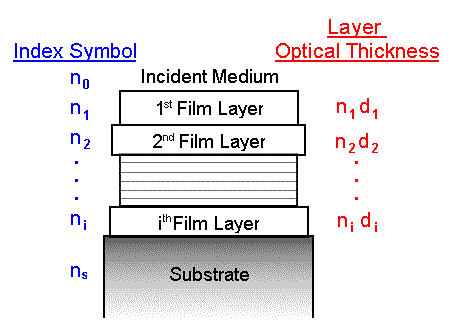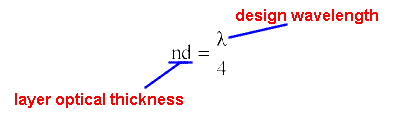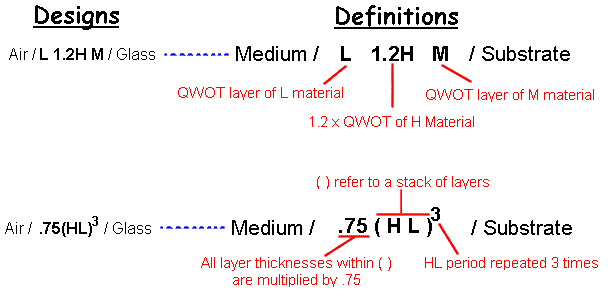Thin Film Design & ApplicationsNomenclature
(symbols, abbreviations, formulas, etc.)

It is important when reading through this course that you, the student, understand all of the symbols, formulas, etc. that are provided within these pages.   The following page lists all of the, thin film language, as it were, that will help you understand the content of this design course.  First let me list a few of the symbols & what they mean:

 n index of refraction of a particular material k extinction coefficient of a particular material (related to absorption ) l wavelength of light (in most cases, the design wavelength) d physical thickness of a thin film layer nd optical thickness of a thin film layerThere is a term that you, the student will see repeated over and over in every design technique given in this course.  That term is a quarterwave optical thickness, or a QWOT.  A QWOT is a specific optical thickness that is defined by the equation below, where l is the design wavelength:All designs in this course will be descibed in terms of QWOT's.  The following diagram outlines how the designs will be notatated:Web www.kruschwitz.com